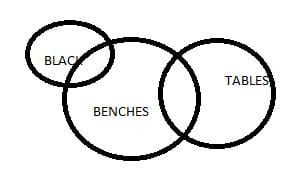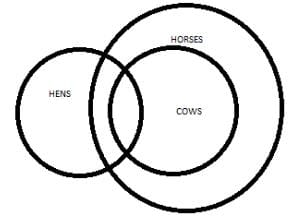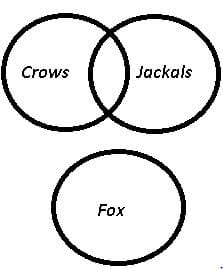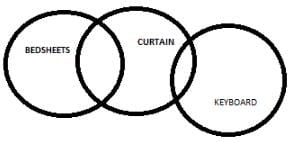CLAT  >  Test: Syllogism- 3

# Test: Syllogism- 3

Test Description

## 20 Questions MCQ Test Logical Reasoning for CLAT | Test: Syllogism- 3

Test: Syllogism- 3 for CLAT 2023 is part of Logical Reasoning for CLAT preparation. The Test: Syllogism- 3 questions and answers have been prepared according to the CLAT exam syllabus.The Test: Syllogism- 3 MCQs are made for CLAT 2023 Exam. Find important definitions, questions, notes, meanings, examples, exercises, MCQs and online tests for Test: Syllogism- 3 below.
Solutions of Test: Syllogism- 3 questions in English are available as part of our Logical Reasoning for CLAT for CLAT & Test: Syllogism- 3 solutions in Hindi for Logical Reasoning for CLAT course. Download more important topics, notes, lectures and mock test series for CLAT Exam by signing up for free. Attempt Test: Syllogism- 3 | 20 questions in 15 minutes | Mock test for CLAT preparation | Free important questions MCQ to study Logical Reasoning for CLAT for CLAT Exam | Download free PDF with solutions
 1 Crore+ students have signed up on EduRev. Have you?
Test: Syllogism- 3 - Question 1

### Directions : In each question below are given two Statements: followed by two conclusion numbered I and II. You have to take the two given statement to be true even if they seem to be at variance with commonly known facts and then decide which of the given Conclusions: logically follows from the two given statement, disregarding commonly know facts. Statements:                       Some benches are tables.              No table is black. Conclusions:                     I. Some tables are benches.              II. No bench is black.

Detailed Solution for Test: Syllogism- 3 - Question 1some tables are benches, and some benches or all benches can be black.

Test: Syllogism- 3 - Question 2

### Directions : In each question below are given two Statements: followed by two conclusion numbered I and II. You have to take the two given statement to be true even if they seem to be at variance with commonly known facts and then decide which of the given Conclusions: logically follows from the two given statement, disregarding commonly know facts. Statements:              All gardens are trees.              Some trees are butterflies. Conclusions:                     I. Some gardens are butterflies.             II. Some butterflies are trees

Test: Syllogism- 3 - Question 3

### Directions : In each question below are given two Statements: followed by two conclusion numbered I and II. You have to take the two given statement to be true even if they seem to be at variance with commonly known facts and then decide which of the given Conclusions: logically follows from the two given statement, disregarding commonly know facts. Statements:                                    All pendrives are chalks.              All chalks are computers. Conclusions:                     I. All computers are pendrives.             II. All pendrives are computers

Test: Syllogism- 3 - Question 4

Directions : In each question below are given two Statements: followed by two conclusion numbered I and II. You have to take the two given statement to be true even if they seem to be at variance with commonly known facts and then decide which of the given Conclusions: logically follows from the two given statement, disregarding commonly know facts.

Statements:

Some children are flowers.

Some flowers are novels.

Conclusions:

I. Some children are novels.

II. No child is a flower.

Test: Syllogism- 3 - Question 5

Directions : In each question below are given two Statements: followed by two conclusion numbered I and II. You have to take the two given statement to be true even if they seem to be at variance with commonly known facts and then decide which of the given Conclusions: logically follows from the two given statement, disregarding commonly know facts.

Statements:

Some hens are cows.

All cows are horses.

Conclusions:

I. Some horses are hens.

II. Some hens are horses

Detailed Solution for Test: Syllogism- 3 - Question 5

The correct option is D.

Both the conclusions can be drawn from the given statement.Test: Syllogism- 3 - Question 6

Directions : In each question below are given two Statements: followed by two conclusion numbered I and II. You have to take the two given statement to be true even if they seem to be at variance with commonly known facts and then decide which of the given Conclusions: logically follows from the two given statement, disregarding commonly know facts.

Statements:

Some poets are producers.

Some producers are trees.

Conclusions:

I. Some poets are trees.

II. Some trees are producers.

Test: Syllogism- 3 - Question 7

Directions : In each question below are given two Statements: followed by two conclusion numbered I and II. You have to take the two given statement to be true even if they seem to be at variance with commonly known facts and then decide which of the given Conclusions: logically follows from the two given statement, disregarding commonly know facts.

Statements:

No pole is a house.

Conclusions:

II. Some houses are poles.

Test: Syllogism- 3 - Question 8

Directions : In each question below are given two Statements: followed by two conclusion numbered I and II. You have to take the two given statement to be true even if they seem to be at variance with commonly known facts and then decide which of the given Conclusions: logically follows from the two given statement, disregarding commonly know facts.

Statements:

All novels are pencils.

All pencils are flowers.

Conclusions:

I. All novels are flowers

II. Some pencils are not book.

Test: Syllogism- 3 - Question 9

Directions : In each question below are given two Statements: followed by two conclusion numbered I and II. You have to take the two given statement to be true even if they seem to be at variance with commonly known facts and then decide which of the given Conclusions: logically follows from the two given statement, disregarding commonly know facts.

Statements:

Some crows are jackals.

No fox is crow.

Conclusions:

I. Some jackals are foxes.

II. Some jackals are not foxes.

Detailed Solution for Test: Syllogism- 3 - Question 9Conclusion:

I. Some jackals are foxes. = may or may not be true

II. Some jackals are not foxes. = may or may not be true

Test: Syllogism- 3 - Question 10

Directions : In each question below are given two Statements: followed by two conclusion numbered I and II. You have to take the two given statement to be true even if they seem to be at variance with commonly known facts and then decide which of the given Conclusions: logically follows from the two given statement, disregarding commonly know facts.

Statements:

Some curtains are bed sheets.

Very few keyboards are curtains.

Conclusions:

I. Some bed sheets are keyboards.

II. No curtains are keyboard.

Detailed Solution for Test: Syllogism- 3 - Question 10

The correct option is CTest: Syllogism- 3 - Question 11

Directions : In each question below are given two Statements: followed by two conclusion numbered I and II. You have to take the two given statement to be true even if they seem to be at variance with commonly known facts and then decide which of the given Conclusions: logically follows from the two given statement, disregarding commonly know facts.

Statements:

All table lamps are electric goods.

Conclusions:

Detailed Solution for Test: Syllogism- 3 - Question 11

C is the correct option. Neither of the conclusions follows because If all radios are electric goods and all table lamps are electric goods there is a possibility that radios might overlap table lamps but here the conclusions are SOME radios are table lamps and SOME table lamps are radios which we can not say clearly.

Test: Syllogism- 3 - Question 12

Directions : In each question below are given two Statements: followed by two conclusion numbered I and II. You have to take the two given statement to be true even if they seem to be at variance with commonly known facts and then decide which of the given Conclusions: logically follows from the two given statement, disregarding commonly know facts.

Statements:

Men are sinners.

Saints are men.

Conclusions:

I. Saints are sinners.

II. Sinners are saints

Test: Syllogism- 3 - Question 13

Directions : In each question below are given two Statements: followed by two conclusion numbered I and II. You have to take the two given statement to be true even if they seem to be at variance with commonly known facts and then decide which of the given Conclusions: logically follows from the two given statement, disregarding commonly know facts.

Statements:

Conclusions:

I. This thief is a man.

II. Some men are thieves.

Detailed Solution for Test: Syllogism- 3 - Question 13

D is the correct option.in this question statement implies that a graduate is a man and a man is a thief. The assumption is this thief would be man or some thieves would be man. So both conclusions are follows.

Test: Syllogism- 3 - Question 14

Directions : In each question below are given two Statements: followed by two conclusion numbered I and II. You have to take the two given statement to be true even if they seem to be at variance with commonly known facts and then decide which of the given Conclusions: logically follows from the two given statement, disregarding commonly know facts.

Statements:

All gases are solids.

All solids are liquids.

Conclusions:

I. All gases are liquids.

II. At least some liquids are solids.

Test: Syllogism- 3 - Question 15

In each of the questions below are given three statements followed by three or more conclusions. You have to take the given statements to be true even if they seem to be at variance with the commonly known facts.

Statements:

Some ducks are canes.

Some canes are books.

All books are lanterns.

Conclusions:

I.   Some lanterns are canes.

II.  Some lanterns are ducks.

III. All canes are lanterns.

IV.  All ducks are lanterns.

Test: Syllogism- 3 - Question 16

In each of the questions below are given three statements followed by three or more conclusions. You have to take the given statements to be true even if they seem to be at variance with the commonly known facts.

Statements:

All markers are black.
All needles are black.
All toys are black.

Conclusions:

I. Some needles are markers.

II.  Some toys are markers.

III. All markers are toys.

IV. All black are markers.

Detailed Solution for Test: Syllogism- 3 - Question 16

The correct answer is D as none of the options given is correct and by drawing the vein diagram .

Test: Syllogism- 3 - Question 17

In each of the questions below are given three statements followed by three or more conclusions. You have to take the given statements to be true even if they seem to be at variance with the commonly known facts.

Statements:

All stores are tents.
Some tents are stones.
All stones are walls.

Conclusions:

I.   Some walls are stores.

II.    Some walls are tents.

III. All stones are stores.

IV.   All walls are stores.

Test: Syllogism- 3 - Question 18

In each of the questions below are given three statements followed by three or more conclusions. You have to take the given statements to be true even if they seem to be at variance with the commonly known facts.

Statements:

Some bricks are gates.
Some gates are roof.
All wheels are bricks.

Conclusions:

I. Some wheels are gates.

II.  No gate is wheel

III.  Some gates are bricks.

IV.  All roof are bricks.

Detailed Solution for Test: Syllogism- 3 - Question 18

D is the correct option. a and b both are possible conclusions.. either a or b and it follows 3 as some bricks are gates therefore there is a clear probability of some gates are bricks.

Test: Syllogism- 3 - Question 19

In each of the questions below are given three statements followed by three or more conclusions. You have to take the given statements to be true even if they seem to be at variance with the commonly known facts.

Statements:

All desks are benches.
No bench is chair.

Conclusions:

II.   No chair is desk.

Detailed Solution for Test: Syllogism- 3 - Question 19

B is the correct option as I and II follow the statement. III and IV don't follow as if all desks are benches and no bench is chair, so none of the chairs can be a desk and also if all chairs are road so some roads can be chairs.

Test: Syllogism- 3 - Question 20

In each of the questions below are given three statements followed by three or more conclusions. You have to take the given statements to be true even if they seem to be at variance with the commonly known facts.

Statements:

All chains are cycles.
Some cycles are wheels.
All wheels are mirrors.

Conclusions:

I.   Some cycles are mirrors.

II.   Some wheels are cycles.

III. Some mirrors are cycles.

IV.   All chains are wheels.

## Logical Reasoning for CLAT

19 videos|27 docs|67 tests
 Use Code STAYHOME200 and get INR 200 additional OFF Use Coupon Code
Information about Test: Syllogism- 3 Page
In this test you can find the Exam questions for Test: Syllogism- 3 solved & explained in the simplest way possible. Besides giving Questions and answers for Test: Syllogism- 3, EduRev gives you an ample number of Online tests for practice

## Logical Reasoning for CLAT

19 videos|27 docs|67 tests